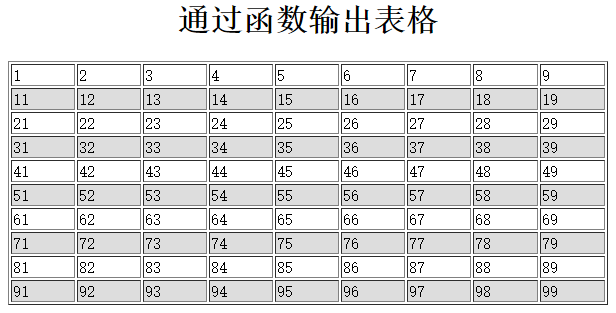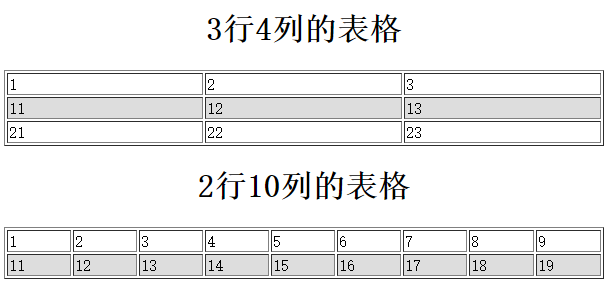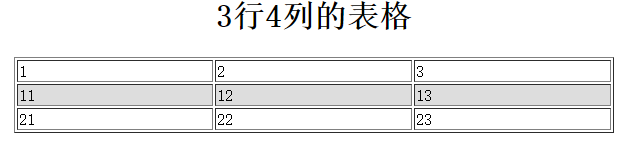PHP学习笔记之PHP的函数应用莎利亚260 0 0

## 一、函数的定义

PHP的模块化程序结构都是通过函数或对象来实现的，函数则是将复杂的PHP程序分为若干个功能模块，每个模块都编写成一个PHP函数，然后通过在脚本中调用函数，以及在函数中调用函数来实现一些大型问题的PHP脚本编写。使用函数的优越性如下所示：

• 提高程序的重用性
• 提高软件的可维护性
• 提高软件的开发效率
• 提高软件的可靠性
• 控制程序设计的复杂性

## 二、自定义函数

PHP中的函数分为系统函数和自定义函数，所谓的系统函数是在PHP中提供的可以直接使用的函数，每一个系统函数都是一个完整的可以完成指定任务的代码段。如果某些功能模块在PHP中没有提供系统函数，就需要自己定义函数。

#### 1. 函数的声明

function 函数名([参数1，参数2，...参数n]){
函数体;
return 返回值
} 

• 每个函数的第一行都是函数头，由声明函数的关键字function、函数名和参数列表三部分组成，其中每一部分完成特定的功能。
• 每个自定义函数都必须使用"function"关键字声明。
• 函数名可以代表整个函数，可以将函数命名为任何名称，只要遵循变量名的命名规则即可。每个函数都有唯一的名称，但需要注意的是，在PHP中不能使用函数重载，所以不能定义重名的函数，也包括不能和系统函数同名。给函数指定一个描述功能的名称是一个不错的主意。
• 声明函数时函数名后面的括号也是必须有的，在括号中表明了一组可以接受的参数列表，参数就是声明的变量，然后在调用函数时传递给它值。参数列表可以没有，也可以有一个或多个参数，多个参数使用逗号隔开。
• 函数体位于函数头后面，用花括号括起来。实际的工作是在函数体中完成的。函数被调用后，首先执行函数中第一条语句，执行到return语句或最外面的花括号后结束，返回到调用的程序。函数体中可以使用任何有效的PHP代码，甚至是其他的函数或类的定义也可以在函数体中声明。
• 使用关键字return可以从函数中返回一个值，在return后面加上一个表达式，程序执行到return语句时，该表达式将被计算，然后返回到调用程序处继续执行。函数的返回值为该表达式的值。

/*将使用双层for循环输出表格的代码声明为函数，函数名为table*/
function table() {                                         //函数名为table
echo "<table align='center' border='1' width='600'>";
echo "<caption><h1>通过函数输出表格</h1></caption>";

for($out=0;$out<10;$out++){ //使用外层循环输出表格行$bgcolor = $out%2 == 0 ? "#FFFFFF" : "#DDDDDD"; //设置隔行变色 echo "<tr bgcolor=".$bgcolor.">";

for($in=1;$in<10;$in++){ //使用内层循环输出表格列 echo "<td>".($out*10+$in)."<td>"; } echo "</tr>"; } echo "</table>"; } table();  输出结果：J)_7QR9T0PF_)G(TB$FW)I.png

#### 2、函数的调用####

• 通过函数名称去调用函数，并让函数体的代码运行，调用几次函数体就会执行几次。
• 如果函数有参数列表，还可以通过函数名后面的小括号传入对应的值给参数，在函数体中使用参数来改变函数内部代码的执行行为。
• 如果函数有返回值，当函数执行完毕时就会将return后面的值返回到调用函数的位置处，这样就可以把函数名称当做函数返回的值使用。

table();
function table(){

}
table(); 

#### 3、函数的参数

/**
* 自定义函数table()时，声明三个参数，参数之间使用逗号隔开
* @param $tableName 需要一个字符串类型的表明 * @param$rows             需要一个整型数值设置表格的行数
* @param $cols 需要一个整型数值设置表格的列数 */ function table($tableName,$rows,$cols){
echo "<table align='center' border='1' width='600'>";
echo "<caption><h1>".$tableName."</h1></caption>"; for($out=0;$out<$rows;$out++){$bgcolor = $out % 2 == 0 ? "#FFFFFF" : "#DDDDDD"; echo "<tr bgcolor=".$bgcolor.">";

for($in=1;$in<$cols;$in++){
echo "<td>".($out*10+$in)."</td>";
}
echo "</tr>";
}
echo "</table>";
}
table("3行4列的表格",3,4);
table("2行10列的表格",2,10);1.png

#### 4、函数的返回值

• return语句可以向函数调用者返回函数体中任意确定的值。
• 将程序控制权返回到调用者的作用域，即退出函数。在函数体中如果执行了return语句，它后面的语句就不会再执行。

function table($tableName,$rows,$cols){$str = "";                                  //声明一个空字符串用来存放表格
str .= "<table align='center' border='1' width='600'>";str .= "<caption><h1>".$tableName."</h1></caption>" for($out=0;$out<$rows;$out++){$bgcolor = $out % 2 == 0 ? "#FFFFFF" : "#DDDDDD";$str .= "<tr bgcolor=".$bgcolor.">"; for($in=1;$in<$cols;$in++){$str .= "<td>".($out*10+$in)."</td>";
}
$str .= "</tr>"; }$str .= "</table>";
}
$str = table("3行4列的表格",3,4); echo$str;2.png

#### 5、函数的工作原理和结构化编程

• 结构化程序更容易编写，因为复杂的编程问题被划分为多个更小的、更简单的任务。每个任务由一个函数完成，而函数中的代码和变量独立于程序的其他部分。通过每次处理一个简单的任务，编程速度将更快。
• 结构化程序更容易测试。如果程序中有一些无法运行的代码，结构化设计则使得将问题缩小到特定的代码段（如特定的函数）。

#### 6、PHP变量的范围####

（1）局部变量
局部变量也成为内部变量，是在函数内部声明的变量，其作用域仅限于函数内部，离开该函数后再使用这种变量是非法的。不仅在函数中声明的变量是局部变量，为声明函数设置的参数因为只能在本函数的内部使用所以也是局部变量。区别在于函数的参数具体数值从函数外部获得（函数被调用时传入的值），而直接在函数中声明的变量只能在函数内部被赋值。但它们的作用域都仅限于函数内部，因为当每次函数被调用时，函数内部的变量才被声明，执行完毕后函数内部的变量都被释放。

function demo($one){$two = 100;
echo "在函数内部执行：$one +$two = ".($one+$two) . "<br/>";
}
demo(100);
echo "在函数外部执行：$one +$two = ".($one+$two);      //不能访问 

（2）全局变量
全局变量也成为外部变量，是在函数外部定义的，它的作用域为从变量定义开始，到本程序文件的末尾。和其他编程语言不通，全局变量不是自动设置为可用的。在PHP中，由于函数可以视为单独的程序片段，所以局部变量会覆盖全局变量的能见度，因此在函数中并无法直接调用全局变量。如下所示：

$a = 100;$b = 100;
/**

**/
function demo(){
$c =$a + $b; echo$c;
}
demo();       //$c不能输出  函数中若要使用全局变量，必须要使用globla关键字定义目标变量，以告诉函数主体此变量为全局变量。如下所示 例一： $a = 100;
$b = 200; function demo(){ global$a,$b;$c = $a +$b;
echo $c; } demo(); //300  例二、 $a = 100;
$b = 200; function demo(){$GLOBALS['c] = $GLOBALS['a'] +$GLOBALS['b'];
}
demo();
echo $c; //300  （3）静态变量 局部变量从存储方式上可以分为动态存储类型静态存储类型。函数中的局部变量，如不专门声明为static存储类型，默认都是动态的分配存储空间的。其中的内部动态变量在函数调用结束后自动释放。如果希望在函数执行后，其内部变量依然保存在内存中，应使用静态变量。在函数执行完毕后，静态变量并不会消失，在所有对该函数的调用之间共享，即在函数再次执行时，静态变量将继续前次的结果继续运算。并且尽在脚本的执行期间函数第一次被调用时被初始化。要声明函数变量为静态的，用关键字static。 代码示例： function test(){ static$a = 0;
echo $a;$a++;
}
test();      //第一次运行，输出0
test();      //第二次运行，输出1
test();      //第三次运行，输出2 

#### 7、声明及应用各种形式的PHP函数

• 引用参数的函数

PHP中默认是按值传递参数，而且函数的参数也属于局部变量，所以即使在函数内部改变参数的值，它也不会改变函数外部的值。函数为子程序，调用函数的程序可以成为子程序。父程序直接传递指定的值或是变量给函数使用。由于所传递的值或变量，与函数里的数值分别存储于不通的内存区块，所以如果函数对所导入的数值做了任何变动，并不会对父程序造成直接影响。如下所示：

按值传递
/**
*@param int $arg 需要一个整型值参数 **/ function test($arg){
$arg = 200; }$var = 100;                  //在父程序中声明一个全局变量$var test($var);                  //调用test函数，并将变量$var的值传给函数的参数$arg
echo  $var; //输出100，$var的值没有发生改变 
按引用传递
function test(&$arg){ //$arg 为引用参数
$arg = 200; }$var = 100;
test($var); echo$var;                     //输出200，$var的值在函数中修改变量$arg时被修改 

for($i=0;$i<count($args);$i++){
echo $args[$i] . "<br/>";
}
}
more_args("one","two","three");

#### 8、回调函数####

1、变量函数
变量函数也称为可变函数。如果一个变量名后面有圆括号，PHP将寻找与变量的值同名的函数，并且将尝试执行它。

function one($a,$b){
return $a +$b;
}
function two($a,$b){
return $a*$b + $a*$b;
}
$result = "one"; echo$result(2,3);                   //输出5 

*/
function filter($func){ for($i=0;$i<=100;$i++){
//将参数变量$func加上一个圆括号$func()，则为调用和变量$func值同名的函数 if($func($i)) continue; echo$i . "<br/>";
}
}

/**
*声明一个函数a,如果参数是3的倍数就返回true,否则返回false
*@param int $num 需要一个整数作为参数 */ function a($num){
return $num%3 == 0; } /** *声明一个函数b，如果参数是一个回文数（翻转后还等于自己的数）返回true,否则返回false */ function b($num){
return $num == strrev($num);
}
filter("a");
echo '------------------<br/>';
filter("b"); 

3、借助call_user_func_array()函数自定义回调函数
虽然可以使用变量函数去声明自己的回调函数，但最多的还是通过call_user_func_array()函数去实现。函数call_user_func_array()时PHP中的内置函数。

function fun($msg1,$msg2){
echo '$msg1=' .$msg1;
echo '<br/>';
echo '$msg2=' .$msg2;
}
/**
*通过系统函数call_user_func_array()调用函数fun(),第一个参数为函数fun()的名称字符串，第二个参数则是一个数组，每个元素之会按顺序传递给调用的fun()函数参数列表中
*/
call_user_func_array("fun","a","b"); 

4、类静态函数和对象的方法回调

/**
*声明一个类Demo，类中声明一个静态的成员方法
*/
class Demo{
static function fun($msg1,$msg2){
echo '$msg1=' .$msg1;
echo '<br/>';
echo '$msg2=' .$msg2;
}
}
/*声明一个类Test，类中声明一个普通的成员方法*/
class Test{
function fun($msg1,$msg2){
echo '$msg1=' .$msg1;
echo '<br/>';
echo '$msg2=' .$msg2;
}
}
/**
通过系统函数call_user_func_array()调用Demo类中的静态成员方法fun(),

*/
call_user_func_array(array("Demo","fun",array("a","b"));
/**
通过系统函数call_user_func_array()调用Test类的实例对象中的成员方法fun(),

*/
call_user_func_array(array(new Test(),"fun"),array("a","b"); 

#### 9、递归函数

函数递归调用最大的好处在于可以精简程序中的繁杂重复调用程序，并且能以这种特性来执行一些较为复杂的运算动作。

function test(){
echo $n . "&nbsp;&nbsp"; if($n>0){
test($n-1); }else{ echo "<-->"; } echo$n . "&nbsp;&nbsp";
} `

#### 10、使用自定义函数库

require()与include()
require()语句的性能与include()相类似，都是包括并运行指定文件。不同之处在于，对include()语句来说，在执行文件时每次都要进行读取和评估；而对于require()语句来说，文件只处理一次（实际上，文件内容替换了require()语句）。这就意味着如果可能执行多次的代码，则使用require()效率比较高。另一方面，如果每次执行代码时是读取不同的文件，或者有通过文件迭代地循环就使用include()语句。JWT 认证方案学习 2021-03-25 22:28